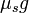# Traction

(diff) ← Older revision | Latest revision (diff) | Newer revision → (diff)

## Definition

Traction refers to the maximum possible value of friction force between a car and the road/ground on which it is running, for which the no slip condition is maintained, i.e., the wheels (of the tires) keep rolling.

It thus represents the maximum value of force that can be applied to accelerate or decelerate the car while maintaining the no slip condition.

## Value of traction

If we assume the Coulomb model of friction, then, on a horizontal road, we have:

Traction = Limiting coefficient of static friction (between the tires and the road)$\times$ Weight of the car.

If the limiting coefficient of static friction is$\mu_s$, the maximum achievable magnitude of acceleration while preserving the no slip condition is (independent of the mass of the car):$\mu_sg$

Here$g$ denotes the value of acceleration due to gravity.

### On an incline

We need to use the model of rolling motion along an inclined plane. Fill this in later